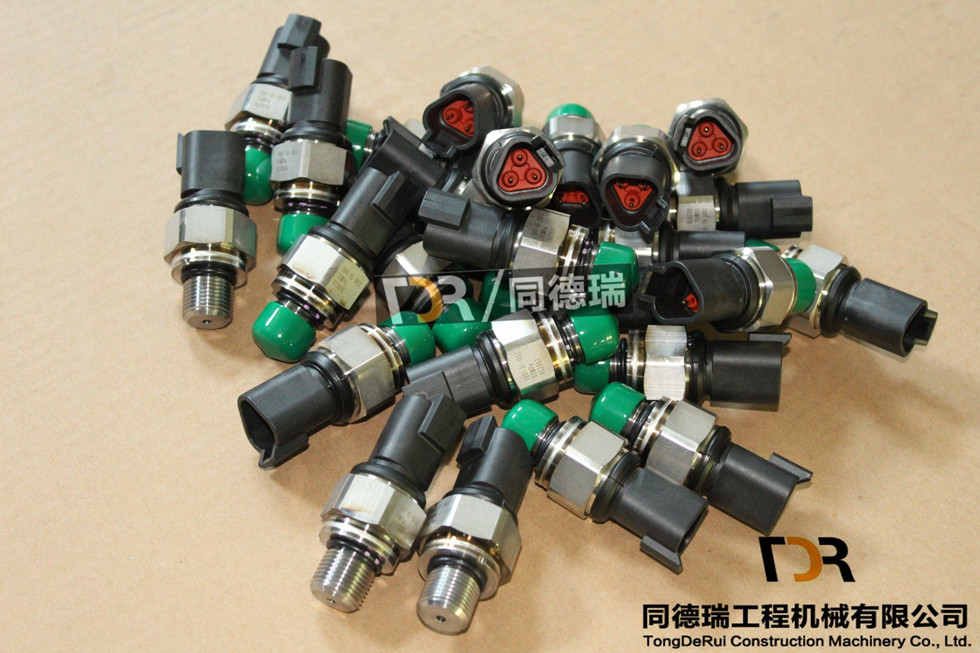# Research and Discussion on Connection Bolts of Hybrid Explosive Devices

By using high-strength bolts, although the strength deficiency can be partially compensated, on the one hand, the impact toughness of the bolt may be lowered, and on the other hand, it is difficult to completely solve the strength problem due to the limitation of the flange structure. In this paper, the combination sections and bolts of the bolted three-stage combined explosion vessel are simplified into rigid bodies and springs respectively, thus obtaining a three-degree-of-freedom spring-mass system. Through the dynamic analysis of this system, it is trying to explore some aspects in improving the stress state of bolts. The force analysis of the coupling bolt mainly comes from the following aspects of the excitation: (1) The axial impact load determines the axial load in the container due to the movement of the explosion shock wave in the container. The main cause of deformation. In the combined explosion vessel with flange bolt connection, the superposition of the explosion shock wave reflection overpressure in the axial projection of the container wall is P(t)=âˆ«sp(r,H,U,t)cosU1ds where: r, H, U They are the surface coordinates of the container head; U1 is the angle between the normal direction of the head and the axial direction of the container. (2) The effect of the container shell on the bolt The vibration of the container shell is a complex vibration with a dense frequency. Under a specific load, the container shell is mainly controlled by several low frequency vibration frequencies. The vibration coupling between the vibration of the casing and the bolt is weak, and basically does not constitute a major influence on the stress state of the coupling bolt. However, the torsional bending vibration generated by the casing still causes the coupling bolt to be unevenly stressed, resulting in a weak link of the bolt structure - the root of the bolt head and the thread generating fatigue cracks. (3) The influence of the natural vibration frequency of the bolt on its strength is equivalent to a homogeneous and isotropic one-dimensional linear elastic body. It can be known from the longitudinal natural vibration analysis of the rod that the bolt length L=0.58m; For steel bolts, the bolt elastic modulus E = 206 GPa and the material density Q = 7800 kg/m3. The resulting natural frequencies of the bolts are approximately 5.3 kHz, 8.6 kHz, 12.9 kHz, respectively. This frequency range is much higher than the response frequency range of the actual impact load of the bolt, and therefore does not constitute a major influence on the stress state of the coupling bolt. The above analysis shows that in the force analysis of the combined explosive container coupling bolt, it is reasonable to consider only the axial impact load of the container on the coupling bolt.

Ignoring the influence of damping, the explosion vessel can be simplified into a three-degree-of-freedom spring-mass system. The three-degree-of-freedom spring-mass system diagram can be written by the simplified model of the system without considering the initial prestress of the bolt. The equation of motion m10m20m3x1x2x3+k1-k10-k1k1+k2-k20-k2k2+k3x1x2x3=P(t)10-1(3) The initial condition is xi(0)=0; dxi(0)dt=0(i= 1,2,3)(4) Equation can be written as matrix form MXb+KX=P(t)(5) where: M is the mass matrix; K is the stiffness matrix; Xb is the acceleration column vector; x is the displacement column vector ;P(t) is the load column vector. Due to the preload force of the bolt, it is assumed that the flange joint always maintains the bolt preload force which varies within a certain range. The element ki=kb+km, kb and km in the stiffness matrix are the bolt and flange stiffness respectively. P(t) is the explosive load acting on the wall. From the theory of explosive mechanics, the wall load can be expressed as an exponentially decreasing excitation function <1>P(t)=P0e-At(tâ‰¥0)(6) : P0 is the axial peak of the explosive load; A is the load attenuation coefficient. Since only the axial deformation and strength of the bolt are analyzed here, the radial force experienced by the cylinder is not considered. Due to the symmetry of the upper and lower flanges and applying the same bolt preload, k1=k2=k. Therefore, equation (3) can be expressed as m10m20m3x1x2x3+k-k0-k2k-k0-kk+k3x1x2x3=P( t) 10-1(7)3 bolt maximum strain estimation x1x2x3=Ue1Ue2Ue3sin(Xit+U)(8) where: Ue3, Ue2, Ue3 are constants; Xi, U are the natural frequency and the initial phase angle, respectively. Substituting equation (8) into equation (7) yields a eigenvalue equation. Three natural frequencies X1, X2, X3, 0 can be solved from the eigenvalue equation

Sensor Contain many Kinds, Such As Pressure Switch, Revolution Sensor, Fuel Sensor, temperature Sensor and so on.

Sensors are devices that sense physical phenomena or changes in the physical state of an object, convert them into signals or data, and Export them. Sensors can deal with various phenomena and objects, including light, sound, temperature, pressure and electricity. Magnetism, distance, velocity, acceleration, angular velocity and so on.Sensor Switch

Sensor Switch,Light Sensor Switch,Occupancy Sensor Switch,Outdoor Light Sensor Switch

Jining Tongderui Construction Machinery Co., Ltd. , http://www.tdr-excavatorparts.com